# Decision Making: Equality and Relational Operators

### Decision Making Equality and Relational Operators

A condition is an expression that can be either true or false. This section introduces a simple version of C#'s if statement that allows an application to make a decision based on the value of a condition. For example, the condition "grade is greater than or equal to 60" determines whether a student passed a test. If the condition in an if statement is true, the body of the if statement executes. If the condition is false, the body does not execute. We will see an example shortly.

Conditions in if statements can be formed by using the equality operators (==, and !=) and relational operators (>, <, >= and <=) summarized in Fig. 3.25. The two equality operators (== and !=) each have the same level of precedence, the relational operators (>, <, >= and <=) each have the same level of precedence, and the equality operators have lower precedence than the relational operators. They all associate from left to right.

Figure 3.25. Equality and relational operators.

(This item is displayed on page 103 in the print version)

Standard algebraic equality and relational operators

C# equality or relational operator

Sample C# condition

Meaning of C# condition

Equality operators

=

==

x == y

x is equal to y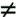!=

x != y

x is not equal to y

Relational operators

>

>

x > y

x is greater than y

<

<

x < y

x is less than y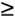>=

x >= y

x is greater than or equal to y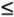<=

x <= y

x is less than or equal to y

The application of Fig. 3.26 uses six if statements to compare two integers entered by the user. If the condition in any of these if statements is true, the assignment statement associated with that if statement executes. The application uses the Console class to prompt for and read two lines of text from the user, extracts the integers from that text with the ToInt32 method of class Convert, and stores them in variables number1 and number2. Then the application compares the numbers and displays the results of the comparisons that are true.

Figure 3.26. Comparing integers using if statements, equality operators and relational operators.

(This item is displayed on pages 103 - 104 in the print version)

``` 1 // Fig. 3.26: Comparison.cs
2 // Comparing integers using if statements, equality operators,
3 // and relational operators.
4 using System;
5
6 public class Comparison
7 {
8 // Main method begins execution of C# application
9 public static void Main( string[] args )
10 {
11 int number1; // declare first number to compare
12 int number2; // declare second number to compare
13
14 //prompt user and read first number
15 Console.Write( "Enter first integer: " );
16 number1 = Convert.ToInt32( Console.ReadLine() );
17
18 //prompt user and read second number
19 Console.Write( "Enter second integer: " );
20 number2 = Convert.ToInt32( Console.ReadLine() );
21
22 if ( number1 == number2 )
23  Console.WriteLine( "{0} == {1}", number1, number2 );
24
25 if ( number1 != number2 )
26  Console.WriteLine( "{0} != {1}", number1, number2 );
27
28 if ( number1 < number2 )
29  Console.WriteLine( "{0} < {1}", number1, number2 );
```
30 31 if ( number1 > number2 ) 32 Console.WriteLine( "{0} > {1}", number1, number2 ); 33 34 if ( number1 <= number2 ) 35 Console.WriteLine( "{0} <= {1}", number1, number2 ); 36 37 if ( number1 >= number2 ) 38 Console.WriteLine( "{0} >= {1}", number1, number2 ); 39 } // end method Main 40 } // end class Comparison
 ```Enter first integer: 42 Enter second integer: 42 42 == 42 42 <= 42 42 >= 42 ```

 ```Enter first integer: 1000 Enter second integer: 2000 1000 != 2000 1000 < 2000 1000 <= 2000 ```

 ```Enter first integer: 2000 Enter second integer: 1000 2000 != 1000 2000 > 1000 2000 >= 1000 ```

The declaration of class Comparison begins at line 6

```public class Comparison
```

The class's Main method (lines 939) begins the execution of the application.

Lines 1112

```int number1; // declare first number to compare
int number2; // declare second number to compare
```

declare the int variables used to store the values entered by the user.

Lines 1416

```// prompt user and read first number
Console.Write( "Enter first integer: " );
```

prompt the user to enter the first integer and input the value. The input value is stored in variable number1.

Lines 18-20

```// prompt user and read second number
Console.Write( "Enter second integer: " );
```

perform the same task, except that the input value is stored in variable number2.

Lines 2223

```if ( number1 == number2 )
Console.WriteLine( "{0} == {1}", number1, number2 );
```

compare the values of the variables number1 and number2 to determine whether they are equal. An if statement always begins with keyword if, followed by a condition in parentheses. An if statement expects one statement in its body. The indentation of the body statement shown here is not required, but it improves the code's readability by emphasizing that the statement in line 23 is part of the if statement that begins in line 22. Line 23 executes only if the numbers stored in variables number1 and number2 are equal (i.e., the condition is true). The if statements in lines 2526, 2829, 3132, 3435 and 3738 compare number1 and number2 with the operators !=, <, >, <= and >=, respectively. If the condition in any of the if statements is true, the corresponding body statement executes.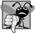## Common Programming Error 3 7

Forgetting the left and/or right parentheses for the condition in an if statement is a syntax errorthe parentheses are required.## Common Programming Error 3 8

Confusing the equality operator, ==, with the assignment operator, =, can cause a logic error or a syntax error. The equality operator should be read as "is equal to," and the assignment operator should be read as "gets" or "gets the value of." To avoid confusion, some people read the equality operator as "double equals" or "equals equals."## Common Programming Error 3 9

It is a syntax error if the operators ==, !=, >= and <= contain spaces between their symbols, as in = =, ! =, > = and < =, respectively.## Common Programming Error 3 10

Reversing the operators !=, >= and <=, as in =!, => and =<, is a syntax error.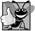## Good Programming Practice 3 12

Indent an if statement's body to make it stand out and to enhance application readability.

Note that there is no semicolon (;) at the end of the first line of each if statement. Such a semicolon would result in a logic error at execution time. For example,

```if ( number1 == number2 ); // logic error
Console.WriteLine( "{0} == {1}", number1, number2 );
```

would actually be interpreted by C# as

```if ( number1 == number2 )
; // empty statement

Console.WriteLine( "{0} == {1}", number1, number2 );
```

where the semicolon in the line by itselfcalled the empty statementis the statement to execute if the condition in the if statement is true. When the empty statement executes, no task is performed in the application. The application then continues with the output statement, which always executes, regardless of whether the condition is true or false, because the output statement is not part of the if statement.## Common Programming Error 3 11

Placing a semicolon immediately after the right parenthesis of the condition in an if statement is normally a logic error.

Note the use of whitespace in Fig. 3.26. Recall that whitespace characters, such as tabs, newlines and spaces, are normally ignored by the compiler. So statements may be split over several lines and may be spaced according to your preferences without affecting the meaning of an application. It is incorrect to split identifiers, strings, and multicharacter operators (like >=). Ideally, statements should be kept small, but this is not always possible.## Good Programming Practice 3 13

Place no more than one statement per line in an application. This format enhances readability.## Good Programming Practice 3 14

A lengthy statement can be spread over several lines. If a single statement must be split across lines, choose breaking points that make sense, such as after a comma in a comma-separated list, or after an operator in a lengthy expression. If a statement is split across two or more lines, indent all subsequent lines until the end of the statement.

Figure 3.27 shows the precedence of the operators introduced in this chapter. The operators are shown from top to bottom in decreasing order of precedence. All these operators, with the exception of the assignment operator, =, associate from left to right. Addition is left associative, so an expression like x + y + z is evaluated as if it had been written as ( x + y ) + z. The assignment operator, =, associates from right to left, so an expression like x = y = 0 is evaluated as if it had been written as x = ( y = 0 ), which, as you will soon see, first assigns the value 0 to variable y and then assigns the result of that assignment, 0, to x.

Figure 3.27. Precedence and associativity of operations discussed.

(This item is displayed on page 107 in the print version)

Operators

Associativity

Type

*

/

%

left to right

multiplicative

+

-

left to right

<

<=

>

>=

left to right

relational

==

!=

left to right

equality

=

right to left

assignment## Good Programming Practice 3 15

Refer to the operator precedence chart (the complete chart is in Appendix A) when writing expressions containing many operators. Confirm that the operations in the expression are performed in the order you expect. If you are uncertain about the order of evaluation in a complex expression, use parentheses to force the order, as you would do in algebraic expressions. Observe that some operators, such as assignment, =, associate from right to left rather than from left to right.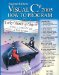Visual C# 2005 How to Program (2nd Edition)
ISBN: 0131525239
EAN: 2147483647
Year: 2004
Pages: 600